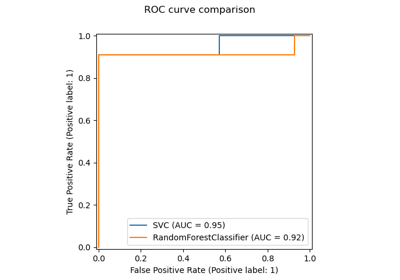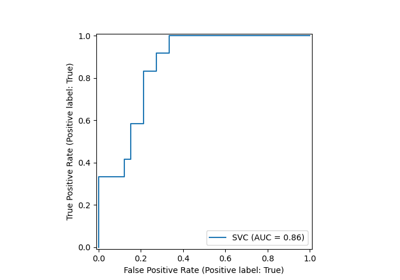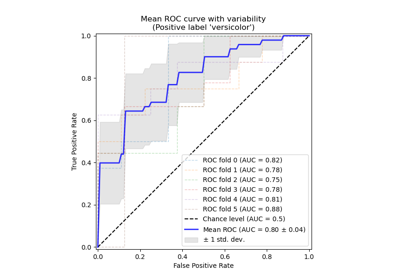# sklearn.metrics.plot_roc_curve¶

sklearn.metrics.plot_roc_curve(estimator, X, y, *, sample_weight=None, drop_intermediate=True, response_method='auto', name=None, ax=None, **kwargs)[source]

Plot Receiver operating characteristic (ROC) curve.

Extra keyword arguments will be passed to matplotlib’s plot.

Read more in the User Guide.

Parameters
estimatorestimator instance

Fitted classifier or a fitted Pipeline in which the last estimator is a classifier.

X{array-like, sparse matrix} of shape (n_samples, n_features)

Input values.

yarray-like of shape (n_samples,)

Target values.

sample_weightarray-like of shape (n_samples,), default=None

Sample weights.

drop_intermediateboolean, default=True

Whether to drop some suboptimal thresholds which would not appear on a plotted ROC curve. This is useful in order to create lighter ROC curves.

response_method{‘predict_proba’, ‘decision_function’, ‘auto’} default=’auto’

Specifies whether to use predict_proba or decision_function as the target response. If set to ‘auto’, predict_proba is tried first and if it does not exist decision_function is tried next.

namestr, default=None

Name of ROC Curve for labeling. If None, use the name of the estimator.

axmatplotlib axes, default=None

Axes object to plot on. If None, a new figure and axes is created.

Returns
displayRocCurveDisplay

Object that stores computed values.

Examples

>>> import matplotlib.pyplot as plt  # doctest: +SKIP
>>> from sklearn import datasets, metrics, model_selection, svm
>>> X, y = datasets.make_classification(random_state=0)
>>> X_train, X_test, y_train, y_test = model_selection.train_test_split(            X, y, random_state=0)
>>> clf = svm.SVC(random_state=0)
>>> clf.fit(X_train, y_train)
SVC(random_state=0)
>>> metrics.plot_roc_curve(clf, X_test, y_test)  # doctest: +SKIP
>>> plt.show()                                   # doctest: +SKIP


## Examples using sklearn.metrics.plot_roc_curve¶